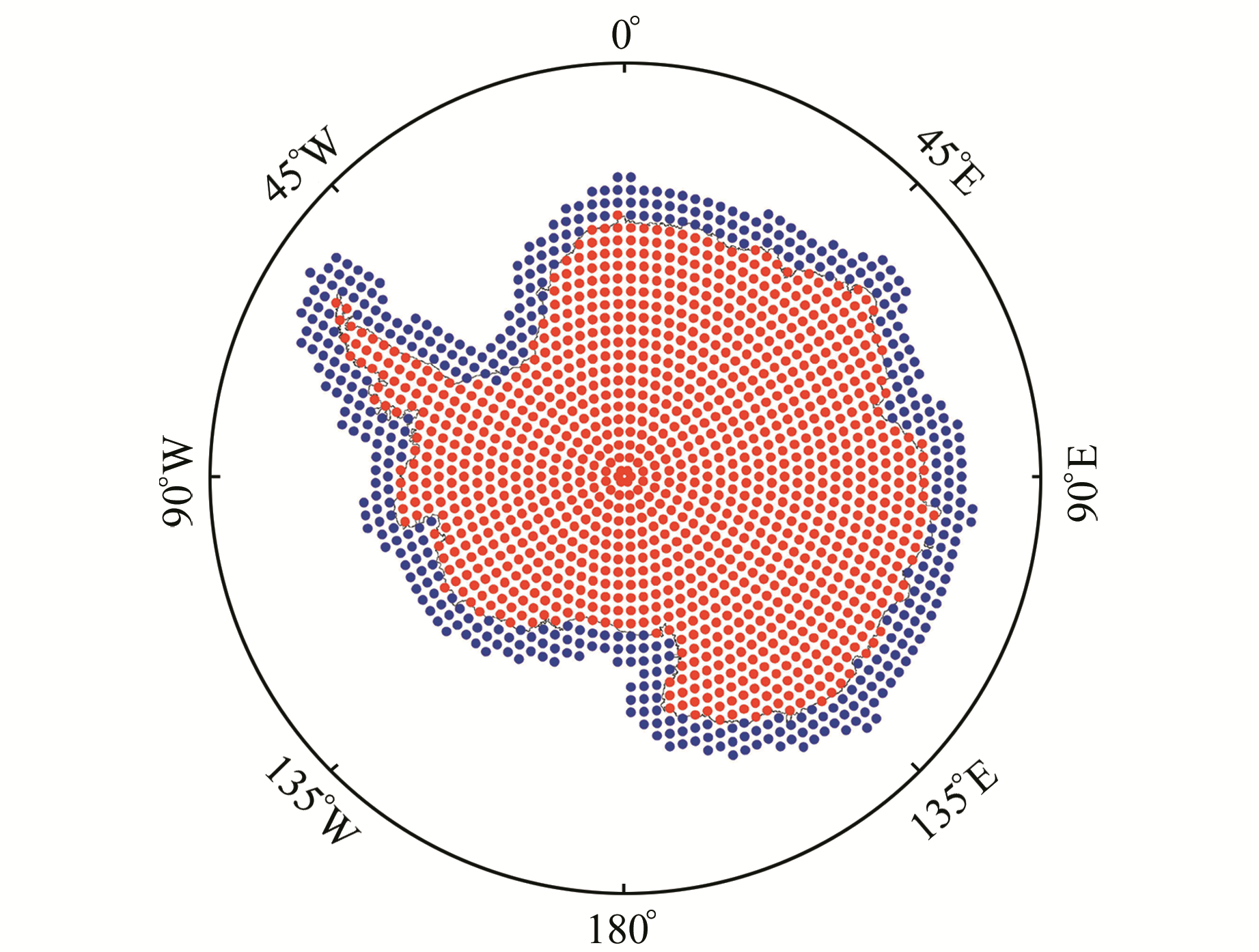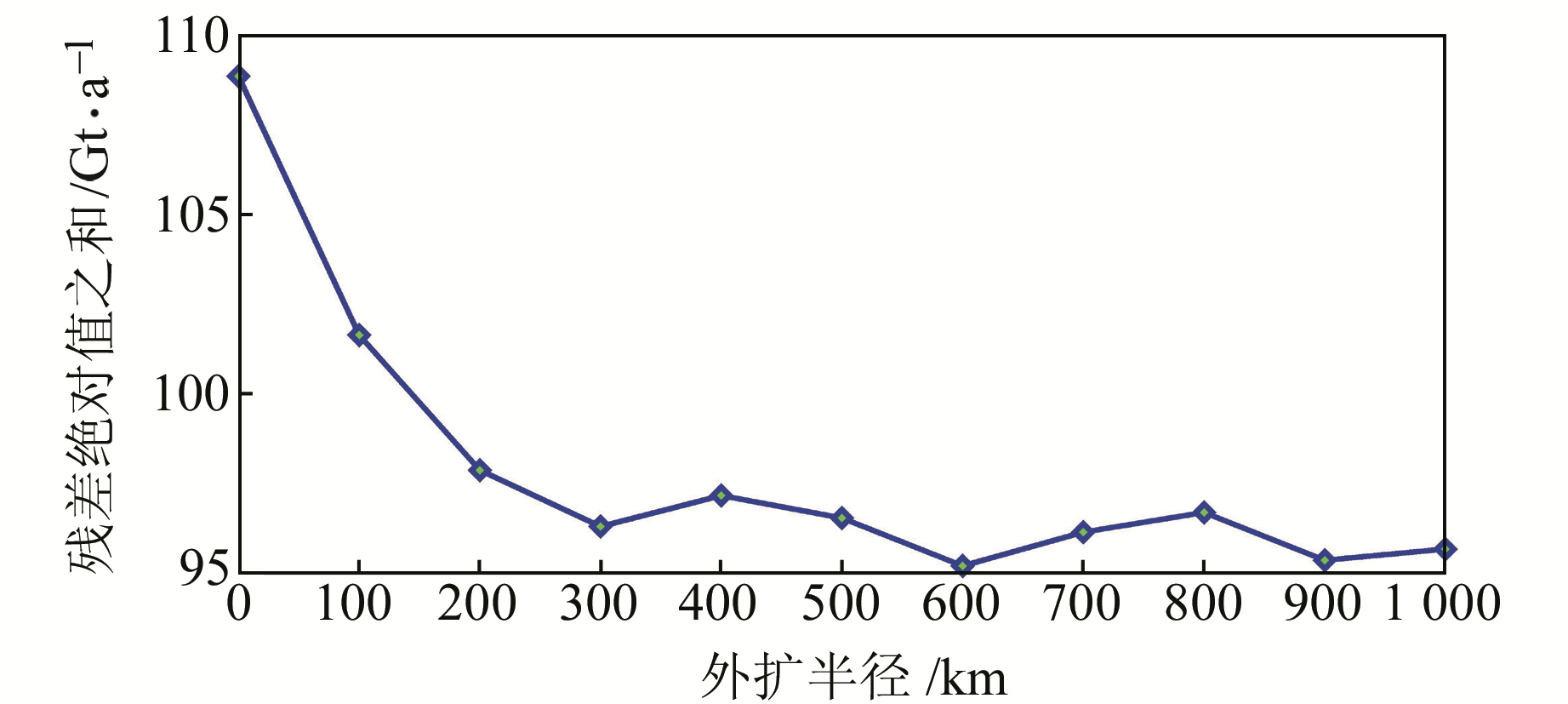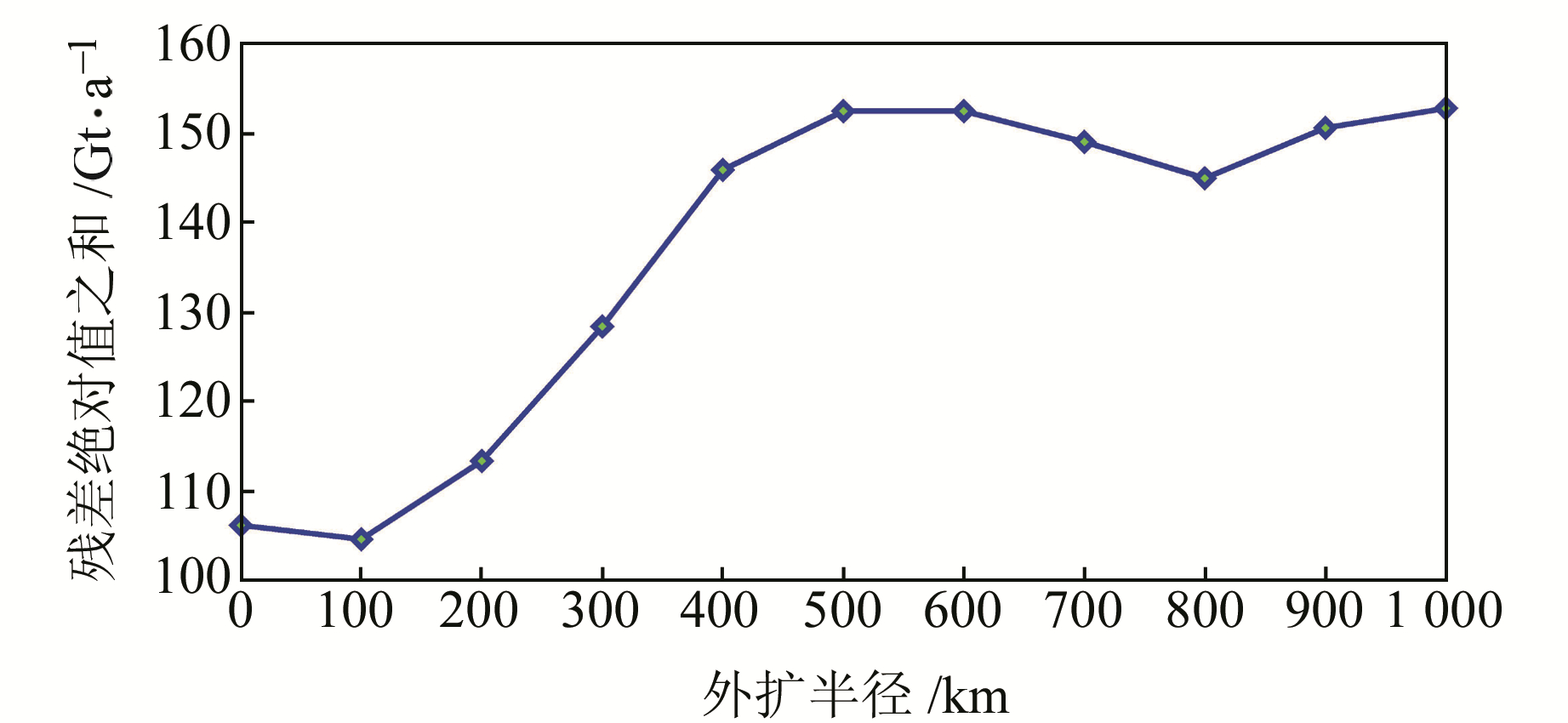﻿ 拟合法改正南极泄露误差最佳外扩边界的确定及其应用文章快速检索 高级检索
 大地测量与地球动力学2019, Vol. 39Issue (11): 1127-1131  DOI: 10.14075/j.jgg.2019.11.006### 引用本文QIU Chunhong, FAN Dongming, GU Yanchao, et al. Estimation and Application of Optimized Extending Range to Reduce[J]. Journal of Geodesy and Geodynamics, 2019, 39(11): 1127-1131.### Foundation support

National Natural Science Foundation of China, No.41574018.

### Corresponding author

FAN Dongming, professor, PhD supervisor, majors in theory and application of satellite gravity measurement, theory and method of precision engineering control survey, E-mail:dongmingfan@163.com.

### 第一作者简介

QIU Chunhong, postgraduate, majors in GRACE application and precision engineering measurement, E-mail:18381670474@163.com.

### 文章历史

1. 西南交通大学地球科学与环境工程学院，成都市高新区西部园区，611756;
2. 中铁二院工程集团有限责任公司，成都市通锦路3号，610031;
3. 高速铁路运营安全空间信息技术国家地方联合工程实验室，成都市高新区西部园区，611756;
4. 西南石油大学土木工程与建筑学院，成都市新都大道8号，610500

GRACE时变重力场模型可反演南极冰盖质量变化，用于研究极地气候变化及冰盖消融对海平面的影响。由于受卫星轨道误差和高频背景模型误差等因素的影响，GRACE时变重力场模型误差随球谐系数阶数的增加而增大，且存在南北条带状噪声[2-3]。目前，大多数研究者采用高斯滤波等空间平滑方法抑制高阶噪声和条带状误差，但空间平滑使地球物理信号泄露至相邻区域，引起泄露误差。Chen等[4-5]提出迭代的全球正向建模恢复法，通过“滤波-修正”迭代算法恢复南极质量变化来改正南极地区的泄露误差；Gunter等将海岸线外扩400 km内的信号当作南极泄露信号，以减小泄露误差的影响；高春春利用自适应边界选取法确定最佳外扩边界，将范围内海域的质量变化归算至南极。Tang等和Mu等采用拟合法确定南极冰盖质量变化，但其将外扩范围内的海洋信号误认为南极泄露信号，影响了南极质量变化的估算。

1 拟合法基本原理

 $\begin{array}{*{20}{l}} {\Delta \bar \sigma ({\theta _j},{\lambda _j}) = \frac{1}{{4\pi }}\sum\limits_{l = 1}^{60} {\;\sum\limits_{m = 0}^l {\;\sum\limits_{i = 1}^I {{\rm{sin}}{\theta _i}\Delta {\theta _i}\Delta {\lambda _i}{{\bar P}_{lm}}({\rm{cos}}{\theta _i})\; \cdot } } } }\\ {\;\;\;\;\;\;\;\;{{\bar P}_{lm}}({\rm{cos}}{\theta _j}){W_l}{\rm{cos}}m({\lambda _i}{\lambda _j})\Delta \sigma ({\theta _i},{\lambda _i})} \end{array}$ (1)

 $\Delta \overline \sigma = {\rm{ }}\mathit{\boldsymbol{A}}\Delta \sigma$ (2)

 $\begin{array}{*{20}{l}} {A\left( {j,i} \right) = \frac{1}{{4\pi }}\sum\limits_{l = 1}^{60} {\;\sum\limits_{m = 0}^l {{\rm{sin}}{\theta _i}\Delta {\theta _i}\Delta {\lambda _i}{{\bar P}_{lm}}({\rm{cos}}{\theta _i}) \cdot } } }\\ {\;\;\;\;\;\;\;\;\;\;{{\bar P}_{lm}}({\rm{cos}}{\theta _j}){W_l}{\rm{cos}}m({\lambda _i}{\lambda _j})} \end{array}$ (3)

 ${\rm{min}}(\left\| {\;\mathit{\boldsymbol{A}}\Delta \sigma - \Delta \bar \sigma \;} \right\|_2^2 + k\left\| {\;\Delta \sigma \;} \right\|_2^2)$ (4)图 1 南极格网划分示意图 Fig. 1 Grid division in Antarctic

 $\Delta \widehat \sigma {^R} = {({\rm{ }}\mathit{\boldsymbol{A}}{^T}\mathit{\boldsymbol{A}}{\rm{ }} + k{\rm{ }}\mathit{\boldsymbol{I}}{\rm{ }})^{1}}\mathit{\boldsymbol{A}}{^T}\Delta \overline \sigma$ (5)

2 模拟实验确定最佳外扩边界 2.1 模拟实验基本方法

2.2 仅考虑南极信号泄露时外扩边界的确定图 2 模拟信号、滤波信号和恢复信号长期变化趋势的空间分布 Fig. 2 Spatial distribution of mass change rate from simulated, filtered, and restored signals表 1 南极区域模拟信号、滤波信号和恢复信号长期变化趋势 Tab. 1 Trend of Antarctic mass change derived from simulated, filtered, and restored signals图 3 恢复信号空间分布一致性指标与外扩半径的关系 Fig. 3 Relationship between the expansion radius and coincidence indicator of spatial distribution of restored mass change
2.3 兼顾海洋信号影响时最佳外扩边界的确定图 4 顾及海洋信号影响时拟合法恢复信号长期变化趋势的空间分布 Fig. 4 Spatial distribution of mass change rate from restored signals when taking the ocean signal into account图 5 顾及海洋信号影响时的恢复信号空间分布一致性指标与外扩半径的关系 Fig. 5 Relationship between the expansion radius and coincidence indicator of spatial distribution of restored mass change when taking the ocean signal into account表 2 顾及海洋信号影响时拟合法恢复信号长期变化趋势 Tab. 2 Mass change derived from restored signals when taking the ocean signal into account

3 GRACE估算南极冰盖质量变化图 6 拟合法恢复CSR RL05时变重力场模型南极质量变化空间分布 Fig. 6 Spatial distribution of Antarctic mass change derived from CSR RL05 data by means of fitting method表 3 拟合法恢复CSR RL05时变重力场模型南极质量变化 Tab. 3 Trend of Antarctic mass change derived from CSR RL05 data by means of fitting method

4 结语

  Schrama E J O, Wouters B, Rietbroek R. A Mascon Approach to Assess Ice Sheet and Glacier Mass Balances and Their Uncertainties from GRACE Data[J]. Journal of Geophysical Research: Solid Earth, 2015, 119(7): 6 048-6 066 (0)  Swenson S, Wahr J. Post-Processing Removal of Correlated Errors in GRACE Data[J]. Geophysical Research Letters, 2006, 33(8) (0)  Wahr J, Swenson S, Velicogna I. Accuracy of GRACE Mass Estimates[J]. Geophysical Research Letters, 2006, 33(6) (0)  Chen J L, Wilson C R, Blankenship D D, et al. Antarctic Mass Rates from GRACE[J]. Geophysical Research Letters, 2006, 33(11) (0)  Chen J L, Wilson C R, Tapley B D. Contribution of Ice Sheet and Mountain Glacier Melt to Recent Sea Level Rise[J]. Nature Geoscience, 2015, 6(7): 549-552 (0)  Gunter B C, Didova O, Riva R E M, et al. Empirical Estimation of Present-Day Antarctic Glacial Isostatic Adjustment and Ice Mass Change[J]. The Cryosphere, 2014, 8(2): 743-760 (0)  高春春.时变重力探测南极冰盖质量平衡的理论、方法与应用研究[D].武汉: 中国科学院测量与地球物理研究所, 2015 (Gao Chunchun. Theory, Method and Application for Detecting Antarctic Ice Sheet Mass Balance from Time-variable Gravity Measurements[D]. Wuhan: Institute of Geodesy and Geophysics, CAS, 2015) (0)  Tang J S, Cheng H W, Liu L. Using Nonlinear Programming to Correct Leakage and Estimate Mass Change from GRACE Observation and Its Application to Antarctica[J]. Journal of Geophysical Research: Solid Earth, 2012, 117(B11) (0)  Mu D P, Yan H W, Feng W, et al. GRACE Leakage Error Correction with Regularization Technique: Case Studies in Greenland and Antarctica[J]. Geophysical Journal International, 2017, 208(3): 1 775-1 786 (0)  邹芳, 金双根. GRACE估计南极冰川质量变化的泄露影响及其改正[J]. 大地测量与地球动力学, 2016, 36(7): 639-644 (Zou Fang, Jin Shuanggen. Effect and Correction of Leakage Errors in Antarctic Glacier[J]. Journal of Geodesy and Geodynamics, 2016, 36(7): 639-644) (0)  Save H, Bettadpur S, Tapley B D. High-Resolution CSR GRACE RL05 Mascons[J]. Journal of Geophysical Research: Solid Earth, 2016, 121(10): 7 547-7 569 DOI:10.1002/2016JB013007 (0)  Swenson S, Chambers D, Wahr J. Estimating Geocenter Variations from a Combination of GRACE and Ocean Model Output[J]. Journal of Geophysical Research: Solid Earth, 2008, 113(B8) (0)  Cheng M K, Ries J C, Tapley B D. Geocenter Variations from Analysis of SLR Data[A]//Altamimi Z. Reference Frames for Applications in Geosciences[M]. Berlin: Springer, 2013 (0)  Geruo A, Wahr J, Zhong S. Computations of the Viscoelastic Response of a 3-D Compressible Earth to Surface Loading: An Application to Glacial Isostatic Adjustment in Antarctica and Canada[J]. Geophysical Journal International, 2013, 192(2): 557-572 DOI:10.1093/gji/ggs030 (0)
Estimation and Application of Optimized Extending Range to Reduce
QIU Chunhong1,2FAN Dongming1,3GU Yanchao4     ZHAO Hongbin1     SU Chunpeng1     YIN Heng1
1. Faculty of Geosciences and Environmental Engineering, Southwest Jiaotong University, West High-Tec Zone, Chengdu 611756, China;
2. China Railway Eryuan Engineering Group Co Ltd, 3 Tongjin Road, Chengdu 610031, China;
3. State-Province Joint Engineering Laboratory of Spatial Information Technology of High-Speed Rail Safety, West High-Tec Zone, Chengdu 611756, China;
4. School of Civil Engineering and Architecture, Southwest Petroleum University, 8 Xindu Road, Chengdu 610500, China
Abstract: In this paper, we use GRACE CSR Mascom data to analyze the effect on the leakage error correctionof boundary expansion and ocean signal within the boundary by means of fitting method in the Antarctic. Simulated results show that the minimum extending range is 300 km, when only considering the leakage-out error from the Antarctic.The ocean signal in the expansion boundary has a significant influence on the Antarctic mass change, particularly for spatial distribution of the mass change along the coastline region. When considering the leakage-out error and the influence of the adjacent ocean signal, the experiment suggests that the optimized extending range is 100 km. Then, the optimized extending range is applied to the fitting method, and the trend of the Antarctic mass change derived from the GRACE CSR RL05 data is -178.61 Gt/a, which is in good agreement with the Mascom results.
Key words: GRACE; Antarctic; leakage error; fitting method; mass balance